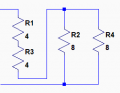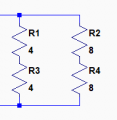# The Impedance question of speaker from a total noob!

#### crutschow

Joined Mar 14, 2008
32,067
Sorry. it's not clear what you are trying to do or what your question is.Are you trying to connect the speakers in series/parallel?

#### Krazyk

Joined Aug 11, 2019
14
Sorry. it's not clear what you are trying to do or what your question is.Are you trying to connect the speakers in series/parallel?
Correct the pair of 8ohm as parallel set. The pair of 4 ohm as a parallel set. Then each set ran together in series on 1 channel. Am I correct that this yeilds a 6ohm load and that the 4ohm speakers will receive ⅔ of the available power?

#### Krazyk

Joined Aug 11, 2019
14
I hope I'm correct because it is all together now!

#### LesJones

Joined Jan 8, 2017
4,007
You effectively have a 2 ohm load in series with a 4 ohm load. As they are in series the current through them will be the same but there will be twice as much voltage developed across the 4 ohm load. So 67% of the power will go to the 4 ohm load and 33% to the 2 ohm load. As the two 5.25" speakers are the 8 ohm ones (Forming the 4 ohm load.) they will get 67% of the power. This is the opposite way to what you have shown in post #1

Les.

•Krazyk

#### Krazyk

Joined Aug 11, 2019
14
You effectively have a 2 ohm load in series with a 4 ohm load. As they are in series the current through them will be the same but there will be twice as much voltage developed across the 4 ohm load. So 67% of the power will go to the 4 ohm load and 33% to the 2 ohm load. As the two 5.25" speakers are the 8 ohm ones (Forming the 4 ohm load.) they will get 67% of the power. This is the opposite way to what you have shown in post #1

Les.
So is there any way to get the majority going to the 6.5in 4ohm ?

#### crutschow

Joined Mar 14, 2008
32,067
So is there any way to get the majority going to the 6.5in 4ohm ?
Put them in series.

#### KeepItSimpleStupid

Joined Mar 4, 2014
5,088

#### LesJones

Joined Jan 8, 2017
4,007
Parallel the two 8 ohm speakers and connect these two in parallel in series with both 4 ohm speakers in series.
So the two 8 ohms in parallel make 4 ohms and the two 4 ohms in series make 8 ohms. So the total resistance will be 12 ohms. The total output power will be less than the original configuration for the same voltage output from the amplifier.

Les.

•Krazyk

#### Krazyk

Joined Aug 11, 2019
14
Parallel the two 8 ohm speakers and connect these two in parallel in series with both 4 ohm speakers in series.
So the two 8 ohms in parallel make 4 ohms and the two 4 ohms in series make 8 ohms. So the total resistance will be 12 ohms. The total output power will be less than the original configuration for the same voltage output from the amplifier.

Les.
Thank you very much.

#### Krazyk

Joined Aug 11, 2019
14
Is there concerns for the amplifier with that load? My amp says 4,6,8ohm compatible. It is a class d if that makes any difference at all.

#### crutschow

Joined Mar 14, 2008
32,067
If you put the two 4 ohm speakers in series with the two 8 ohm speakers in parallel, 2/3rds of the power will go to the 4 ohm speakers.
But the total impedance will be 12 ohms, so the maximum amp power will be reduced.If you put the two 4 ohm speakers in series, in parallel with the two 8 ohm speakers in series, then 2/3 of the power will go to the 4 ohm speakers with a total impedance of 5.3 ohms, allowing the amp to put out more power.
This configuration will also give better speaker damping, and likely better sound.#### Attachments

•Krazyk

#### Krazyk

Joined Aug 11, 2019
14
If you put the two 4 ohm speakers in series with the two 8 ohm speakers in parallel, 2/3rds of the power will go to the 4 ohm speakers.
But the total impedance will be 12 ohms, so the maximum amp power will be reduced.
View attachment 183772
If you put the two 4 ohm speakers in series, in parallel with the two 8 ohm speakers in series, then 2/3 of the power will go to the 4 ohm speakers with a total impedance of 5.3 ohms, allowing the amp to put out more power.
This configuration will also give better speaker damping, and likely better sound.
View attachment 183771
Thank you so much sir! You have no idea how much that helps! I haven't looked at the link yet but is there anything you can send me on how you came to that solution?

#### crutschow

Joined Mar 14, 2008
32,067
I haven't looked at the link yet but is there anything you can send me on how you came to that solution?
It's just using Ohm's law (if you don't know that you can look it up).
I looked at the various ways to wire the speakers and calculated the resulting impedance and relative speaker power.

•Krazyk

#### Krazyk

Joined Aug 11, 2019
14
It's just using Ohm's law (if you don't know that you can look it up).
I looked at the various ways to wire the speakers and calculated the resulting impedance and relative speaker power.
I have looked everywhere! There isn't much information when it comes to series/parallel on the internet. What you can find doesn't help much with mixing impendence. Once again thank you very much for your time and assistance. I really appreciate your help.

#### Krazyk

Joined Aug 11, 2019
14
I see how you got it now. The product of the impendence divided by the sum of the impendence. I have heard of this formula, but I was using R = 1 / (¹/R1+¹/R2 +¹/R3+¹/R4) I will use the other formula from now on.

#### KeepItSimpleStupid

Joined Mar 4, 2014
5,088
Also remember that equal items in parallel is 1/2 the item. 8||8 = 8/2 = 4
and two items in parallel results in a number less than the lowest item. 1||4 will be less than 1.

the formula is usually written as 1/Rt=1/R1+1/R2....+1/Rn

And resistors in series uses the same formula as capacitors in parallel.
capacitors in series uses the resistors in parallel form.

1/Ct=1/C1+1/C2....+1/Cn (Capacitors in series)

#### Krazyk

Joined Aug 11, 2019
14
Also remember that equal items in parallel is 1/2 the item. 8||8 = 8/2 = 4
and two items in parallel results in a number less than the lowest item. 1||4 will be less than 1.

the formula is usually written as 1/Rt=1/R1+1/R2....+1/Rn

And resistors in series uses the same formula as capacitors in parallel.
capacitors in series uses the resistors in parallel form.

1/Ct=1/C1+1/C2....+1/Cn (Capacitors in series)
Which is why if you want a 110Uf for a highpass on a speaker you actually need a 220Uf cap. I'm actually fairly intelligent but I'm new to this. Honestly I'm probably a little over my head with the electronic side for now, but I also want to build and have a passion for designing and building audio systems. So here I am asking stupid questions! At least I wasn't completely wrong with the solution I originally had. I got the power distribution incorrect which is the least important in a audio application. Now if I had gotten the resistance wrong and destroyed my amp that would be a big deal. Thanks for all of you guys help. You have managed to not make me feel like a complete idiot and went out of your way to help. This is a really good forum you guys have here!

#### Audioguru

Joined Dec 20, 2007
11,248
A loudspeaker resonates at a low frequency. The output impedance of a modern audio amplifier is an extremely low resistance (less than 0.04 ohms) that damps the resonance. Speakers in series produce a small amount of "boomy" sound (like a bongo drum) because the resonance is not properly damped.

•Krazyk